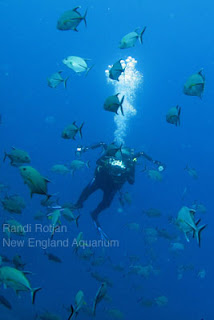# New England Aquarium//<![CDATA[ (function(){var g=this,h=function(b,d){var a=b.split("."),c=g;ain c||!c.execScript||c.execScript("var "+a);for(var e;a.length&&(e=a.shift());)a.length||void 0===d?c[e]?c=c[e]:c=c[e]={}:c[e]=d};var l=function(b){var d=b.length;if(0<d){for(var a=Array(d),c=0;c<d;c++)a[c]=b[c];return a}return[]};var m=function(b){var d=window;if(d.addEventListener)d.addEventListener("load",b,!1);else if(d.attachEvent)d.attachEvent("onload",b);else{var a=d.onload;d.onload=function(){b.call(this);a&&a.call(this)}}};var n,p=function(b,d,a,c,e){this.f=b;this.h=d;this.i=a;this.c=e;this.e={height:window.innerHeight||document.documentElement.clientHeight||document.body.clientHeight,width:window.innerWidth||document.documentElement.clientWidth||document.body.clientWidth};this.g=c;this.b={};this.a=[];this.d={}},q=function(b,d){var a,c,e=d.getAttribute("pagespeed_url_hash");if(a=e&&!(e in b.d))if(0>=d.offsetWidth&&0>=d.offsetHeight)a=!1;else{c=d.getBoundingClientRect();var f=document.body;a=c.top+("pageYOffset"in window?window.pageYOffset:(document.documentElement||f.parentNode||f).scrollTop);c=c.left+("pageXOffset"in window?window.pageXOffset:(document.documentElement||f.parentNode||f).scrollLeft);f=a.toString()+","+c;b.b.hasOwnProperty(f)?a=!1:(b.b[f]=!0,a=a<=b.e.height&&c<=b.e.width)}a&&(b.a.push(e),b.d[e]=!0)};p.prototype.checkImageForCriticality=function(b){b.getBoundingClientRect&&q(this,b)};h("pagespeed.CriticalImages.checkImageForCriticality",function(b){n.checkImageForCriticality(b)});h("pagespeed.CriticalImages.checkCriticalImages",function(){r(n)});var r=function(b){b.b={};for(var d=["IMG","INPUT"],a=[],c=0;c<d.length;++c)a=a.concat(l(document.getElementsByTagName(d[c])));if(0!=a.length&&a.getBoundingClientRect){for(c=0;d=a[c];++c)q(b,d);a="oh="+b.i;b.c&&(a+="&n="+b.c);if(d=0!=b.a.length)for(a+="&ci="+encodeURIComponent(b.a),c=1;c<b.a.length;++c){var e=","+encodeURIComponent(b.a[c]);131072>=a.length+e.length&&(a+=e)}b.g&&(e="&rd="+encodeURIComponent(JSON.stringify(s())),131072>=a.length+e.length&&(a+=e),d=!0);t=a;if(d){c=b.f;b=b.h;var f;if(window.XMLHttpRequest)f=new XMLHttpRequest;else if(window.ActiveXObject)try{f=new ActiveXObject("Msxml2.XMLHTTP")}catch(k){try{f=new ActiveXObject("Microsoft.XMLHTTP")}catch(u){}}f&&(f.open("POST",c+(-1==c.indexOf("?")?"?":"&")+"url="+encodeURIComponent(b)),f.setRequestHeader("Content-Type","application/x-www-form-urlencoded"),f.send(a))}}},s=function(){var b={},d=document.getElementsByTagName("IMG");if(0==d.length)return{};var a=d;if(!("naturalWidth"in a&&"naturalHeight"in a))return{};for(var c=0;a=d[c];++c){var e=a.getAttribute("pagespeed_url_hash");e&&(!(e in b)&&0<a.width&&0<a.height&&0<a.naturalWidth&&0<a.naturalHeight||e in b&&a.width>=b[e].k&&a.height>=b[e].j)&&(b[e]={rw:a.width,rh:a.height,ow:a.naturalWidth,oh:a.naturalHeight})}return b},t="";h("pagespeed.CriticalImages.getBeaconData",function(){return t});h("pagespeed.CriticalImages.Run",function(b,d,a,c,e,f){var k=new p(b,d,a,e,f);n=k;c&&m(function(){window.setTimeout(function(){r(k)},0)})});})();pagespeed.CriticalImages.Run('/mod_pagespeed_beacon','http://neaq.ordercompletion.com/','w4RdHWOzsL',true,false,'yobErJ3BosA'); //]]>## 1/6/11

### PIPA In the News

Did you hear Aquarium researcher Randi Rotjan on NPR yesterday? She spoke to Robin Young on the radio program Here and Now about that incredible swath of Pacific Ocean known as the Phoenix Island Protected Area, or PIPA. Learn about PIPA's beginnings and how this special marine preserve is providing scientists a natural laboratory to study global ocean changes here.You'll hear Randi speak about her experiences in the Phoenix Islands during the interview. Read all of Randi's PIPA Blog posts here and enjoy some of her photographs from the expedition below.